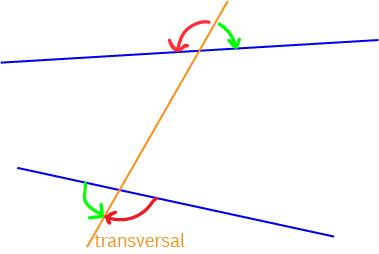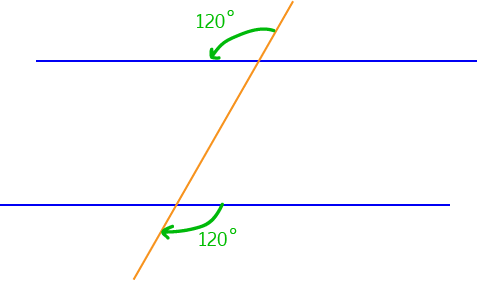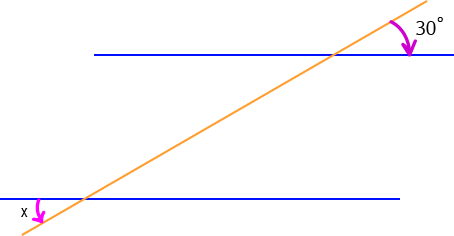# Alternate Exterior Angles

A transversal is a line that intersects with two or more other lines.

When transversals cut lines, they form many different pairs of angles. In this article, we're interested in alternate exterior angles.

## Locating Alternate Exterior AnglesIn the picture, the two lines are blue, and the transversal is orange. Alternate angles lie outside the two lines, but on opposite sides of the transversal. The picture shows two pairs of alternate angles, one marked by red arrows, and the other marked by green arrows.

## Alternate Exterior Angles and Parallel Lines

If the transversal cuts a pair of parallel lines, the alternate exterior angles that are formed are equalIn the picture, the two blue lines are parallel, so the transversal forms equal alternate angles. Both alternate exterior angles marked in green are equal to $60^\circ$.In the picture, the two blue lines are parallel, so the transversal forms equal alternate exterior angles. Both alternate exterior angles marked in green are equal to $120^\circ$.In the picture, the two blue lines are parallel, so the transversal forms equal alternate exterior angles. The angles marked in pink are alternate exterior angles, so they must be equal, and $x$ must equal $30^\circ$.

## A Test for Parallel Lines

Two lines, cut by a transversal, are parallel if the alternate exterior angles are equal. So, if I hadn't told you that the two blue lines in this picture were parallel, you could work it out because the two alternate exterior angles marked are equal.Having equal alternate exterior angles is a test for parallel lines. If the alternate exterior angles aren't equal, then the lines aren't parallel. If the alternate exterior angles are equal, then the lines must be parallel.

### Description

In this mini book, you will learn about

1. Alternate angles
2. Cointerior angles
4. Vertically Opposite Angles

and several other topics related to lines and angles.

### Audience

Year 10 or higher

### Learning Objectives

To learn the basics of Lines and Angles stream of Geometry

Author: Subject Coach
You must be logged in as Student to ask a Question.